# GATE CS 2021 | Set 1

• Last Updated : 17 Sep, 2021

 Question 1
The ratio of boys to girls in a class is 7 to 3. Among the options below, an acceptable value for the total number of students in the class is:
 A 21 B 37 C 50 D 73
GATE CS 2021 | Set 1    General Aptitude    Set Theory & Algebra
Discuss it

Question 1 Explanation:
Given, Ratio of boys to girls = 7 : 3 So, ratio of boys in the class = 7 / 10 = should be integer And, ratio of girls in the class = 3 / 10 = should be integer Hence, from given option, only 50 satisfies = 35 : 15 = 7 : 3 Alternative Method : Sum = 3+7 = 10k So, total number of students should be multiple of 10.
 Question 2
A polygon is convex if, for every pair of points, P and Q belonging to the polygon, the line segment PQ lies completely inside or on the polygon. Which one of the following is NOT a convex polygon?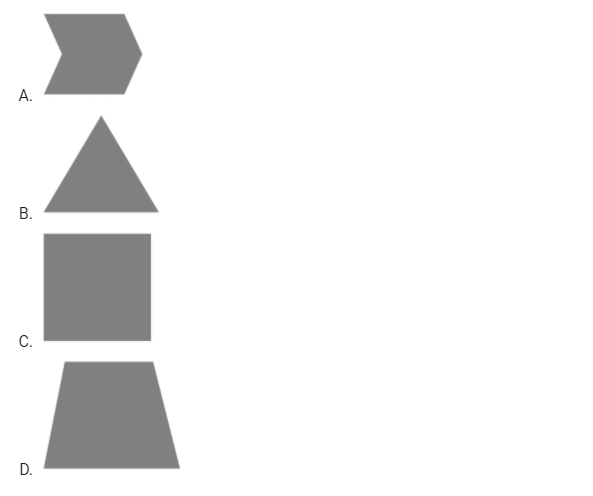A A B B C C D D
GATE CS 2021 | Set 1    General Aptitude
Discuss it

Question 2 Explanation:
It these any two points are inside in polygon, then line by joining these points will also be inside same polygon. Polygon in first image is not a convex because if you take any two corner points then it will not be inside the polygon.
 Question 3
Consider the following sentences:
• (i) Everybody in the class is prepared for the exam.
• (ii) Babu invited Danish to his home because he enjoys playing chess.
Which of the following is the CORRECT observation about the above two sentences?
 A (i) is grammatically correct and (ii) is unambiguous B (i) is grammatically incorrect and (ii) is unambiguous C (i) is grammatically correct and (ii) is ambiguous D (i) is grammatically incorrect and (ii) is ambiguous
GATE CS 2021 | Set 1    English
Discuss it

Question 3 Explanation:
Statement (i) is grammatically correct, (everybody is singular which uses singular verb). Statement (ii) is ambiguous because if "Babu invited Danish to his home and he enjoys playing chess" that does not mean Danish also enjoys playing chess. His purpose of invitation is not clear.
 Question 4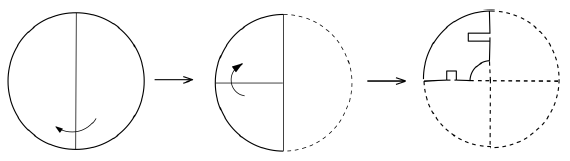A circular sheet of paper is folded along the lines in the direction shown. The paper, after being punched in the final folded state as shown and unfolded in the reverse order of folding, will look like _______.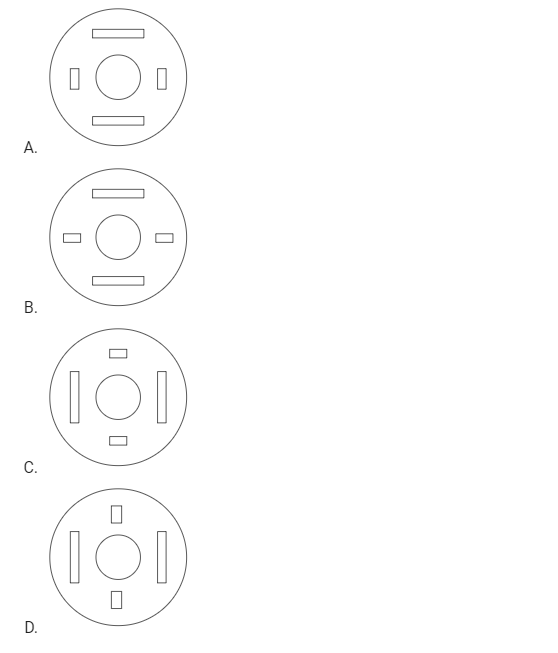A A B B C C D D
GATE CS 2021 | Set 1    General Aptitude
Discuss it

Question 4 Explanation:
If you observe the last image, you can notice that :
• Pillar in Y-axis is longer than pillar in X-axis
• Pillar in Y is horizontal and pillar in Y is vertical
Image given in option (A) satisfied these conditions.
 Question 5
___________ is to surgery as writer is to ___________ Which one of the following options maintains a similar logical relation in the above sentence?
 A Plan, outline B Hospital, library C Doctor, book D Medicine, grammar
GATE CS 2021 | Set 1    English
Discuss it

Question 5 Explanation:
Doctor does surgery as writer writes book.
 Question 6
We have 2 rectangular sheets of paper, M and N, of dimensions 6 cm × 1 cm each. Sheet M is rolled to form an open cylinder by bringing the short edges of the sheet together. Sheet N is cut into equal square patches and assembled to form the largest possible closed cube. Assuming the ends of the cylinder are closed, the ratio of the volume of the cylinder to that of the cube is _________.
 A π/2 B 3/π C 9/π D 3π
GATE CS 2021 | Set 1    General Aptitude
Discuss it

Question 6 Explanation:
Sheet first M is 6x1 size, in which cylinder will be formed by bringing the short edges of the sheet together. Given that ends of the cylinder are closed. So, volume of cylinder (Vcylinder) = π.r2.h Here, circumference = 2πr = 6 So, r = 3/π and h = 1 Substitute in above equation, = volume of cylinder (Vcylinder) = π.(3/π)2.1 = π.(9/π2).1 = 9/π Now, second sheet N is 6x1 size, which cut into equal square patches and assembled to form the largest possible closed cube. So, there would be 6 unit size square which formed a cube which would have unit length (i.e.,) of each side. So, volume of cube (Vcube) = a3 = 1.1.1 = 1 Therefore, the ratio of the volume of the cylinder to that of the cube is = (9/π) / 1 = 9/π Option (C) is correct.
 Question 7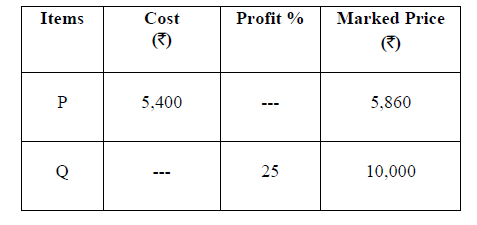Details of prices of two items P and Q are presented in the above table. The ratio of cost of item P to cost of item Q is 3:4. Discount is calculated as the difference between the marked price and the selling price. The profit percentage is calculated as the ratio of the difference between selling price and cost, to the cost
`Profit% = ((Selling price – Cost)/Cost)×100 `
The discount on item Q, as a percentage of its marked price, is _______ .
 A 25 B 12.5 C 10 D 5
GATE CS 2021 | Set 1    General Aptitude    Ratio and Proportion
Discuss it

Question 7 Explanation:
Given, ratio of cost of item P to cost of item Q is 3:4 So, (3/7)*(total cost of P and Q) = 5400 = cost of P Total cost of P and Q = 5400*7/3 = 12600 Hence, cost of Q = 12600*4/7 = 7200 Now, the selling price of Q would be = cost price * (1+profit%) ;as given in table = 7200 * (1+0.25) = 7200*1.25 = 9000 (Discount is calculated as the difference between the marked price and the selling price; as given in table.). Discount % = (MP - SP) / MP = (10000 - 9000) / 10000 = 1000/10000 = 1/10 = 0.1 = 10%
 Question 8
There are five bags each containing identical sets of ten distinct chocolates. One chocolate is picked from each bag. The probability that at least two chocolates are identical is __________ .
 A 0.3024 B 0.4235 C 0.6976 D 0.8125
GATE CS 2021 | Set 1    General Aptitude    Probability    50 Aptitude & Reasoning MCQs with Answers
Discuss it

Question 8 Explanation:
Bags are identical but not chocolates. Chocolates are distinct. We picked one chocolates from each bag. Order does not matter here. The probability that at least two chocolates are identical = 1 - (all are distinct chocolates) = 1 - (10/10*9/10*8/10*7/10*6/10) = 1 - 30240 / 100000 = 69760 / 100000 = 0.6976
 Question 9
Given below are two statements 1 and 2, and two conclusions I and II
• Statement 1: All bacteria are microorganisms.
• Statement 2: All pathogens are microorganisms.
• Conclusion I: Some pathogens are bacteria.
• Conclusion II: All pathogens are not bacteria.
Based on the above statements and conclusions, which one of the following options is logically CORRECT?
 A Only conclusion I is correct B Only conclusion II is correct C Either conclusion I or II is correct D Neither conclusion I nor II is correct
GATE CS 2021 | Set 1    General Aptitude    Set Theory & Algebra
Discuss it

Question 9 Explanation:
We have to find counter example for both given Conclusion, if possible.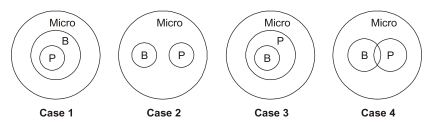• Case-1 is counter example of Conclusion II, because of all pathogens are bacteria in this diagram. Conclusion I would be true in this case, because all so also some. (So, Conclusion I is true and II is false.).
• Case-2 is counter example of Conclusion I, because no pathogens are bacteria. Conclusion II would be true in this case, because all so also some. (So, Conclusion II is false and II is true.).
• Case-3: Conclusion I is true, and Conclusion II is false as some pathogens are *already* bacteria.
• Case-4: Conclusion I is true, and Conclusion II is false as some pathogens are *already* bacteria.
So, we found counter example for both Conclusion. Hence, option (D) is true (Official Key given by GATE 2021.). Important : Note that if we consider any image at any point of time or any same domain, then "Either conclusion I or II is correct" as you can also noticed in any given Venn diagram.
 Question 10
Some people suggest anti-obesity measures (AOM) such as displaying calorie information in restaurant menus. Such measures sidestep addressing the core problems that cause obesity: poverty and income inequality. Which one of the following statements summarizes the passage?
 A The proposed AOM addresses the core problems that cause obesity B If obesity reduces, poverty will naturally reduce, since obesity causes poverty C AOM are addressing the core problems and are likely to succeed D AOM are addressing the problem superficially
GATE CS 2021 | Set 1    English
Discuss it

Question 10 Explanation:
The problems mentioned are poverty and inequality. But AOMs are not addressing the main problems directly. But it is a kind of site step taken to address obesity. So AOMs are only addressing the obesity problem superficially but not addressing the real problems.
There are 65 questions to complete.
My Personal Notes arrow_drop_up